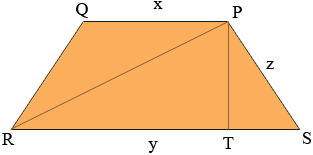SEARCH HOMEMath Central Quandaries & QueriesQuestion from hazel, a student: how to solve the diagonals of an isosceles trapezoid? what is the formula?Hazel,

If you know the lengths of the sides you can use Pythagoras theorem twice to determine the lengths of the diagonals.I let the lengths of the parallel sixes be x and y units with y > x. The two equal length sides have length z. PT is perpendicular to PT.

|TS| = (y - x)/2 and |PS| = z and hence you can use Pythagoras theorem to find |PT|. |RT| = y - |TS| and thus you can use Pythagoras theorem again to find |RP|.

HarleyMath Central is supported by the University of Regina and The Pacific Institute for the Mathematical Sciences.